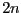Next: Binomial Up: Miscellaneous procedures Previous: Square adjustment   Contents

Box reduction

Let Sol be a solution of a system that has been found in the box B while the algorithm was processing a list of boxes BOX. It is of interest to treat the box and the list of boxes in such way that Sol is no more included in the set of boxes i.e. to have in the list of boxes only the complementary of each box with respect to the box Sol. Note that for a problem withunknowns this complementary may be described by up toboxes.

This may be obtained by using:

int Sol_Reduction(int Dimension,int Dimension_Eq,
INTERVAL_MATRIX &BOX,
INTERVAL_VECTOR &B, INTERVAL_VECTOR &Sol,
int *nb,int type,
int (* Simp_Proc)(INTERVAL_VECTOR &))
where
• nb: the total number of boxes in the list BOX
• type: allow to create at most type new boxes that will be appended to BOX
• Simp_Proc: a simplification procedure for the system
This algorithm will return -1 if the solution box covers completely the box B. Note that this algorithm may create new boxes but only ALIAS_Allows_N_New_Boxes are allowed to be created (with a default value of 2). A similar procedure exists when gradient boxes have also to be stored:

int Sol_Reduction(int Dimension_Var,int Dimension_Eq,
INTERVAL_MATRIX &BBOX,
INTERVAL_MATRIX &GBBOX,
INTERVAL_VECTOR &P, INTERVAL_VECTOR &PP1,
int current_box,int *nb_box,
int nb_max_box,int type,
int (* Simp_Proc)(INTERVAL_VECTOR &))
where